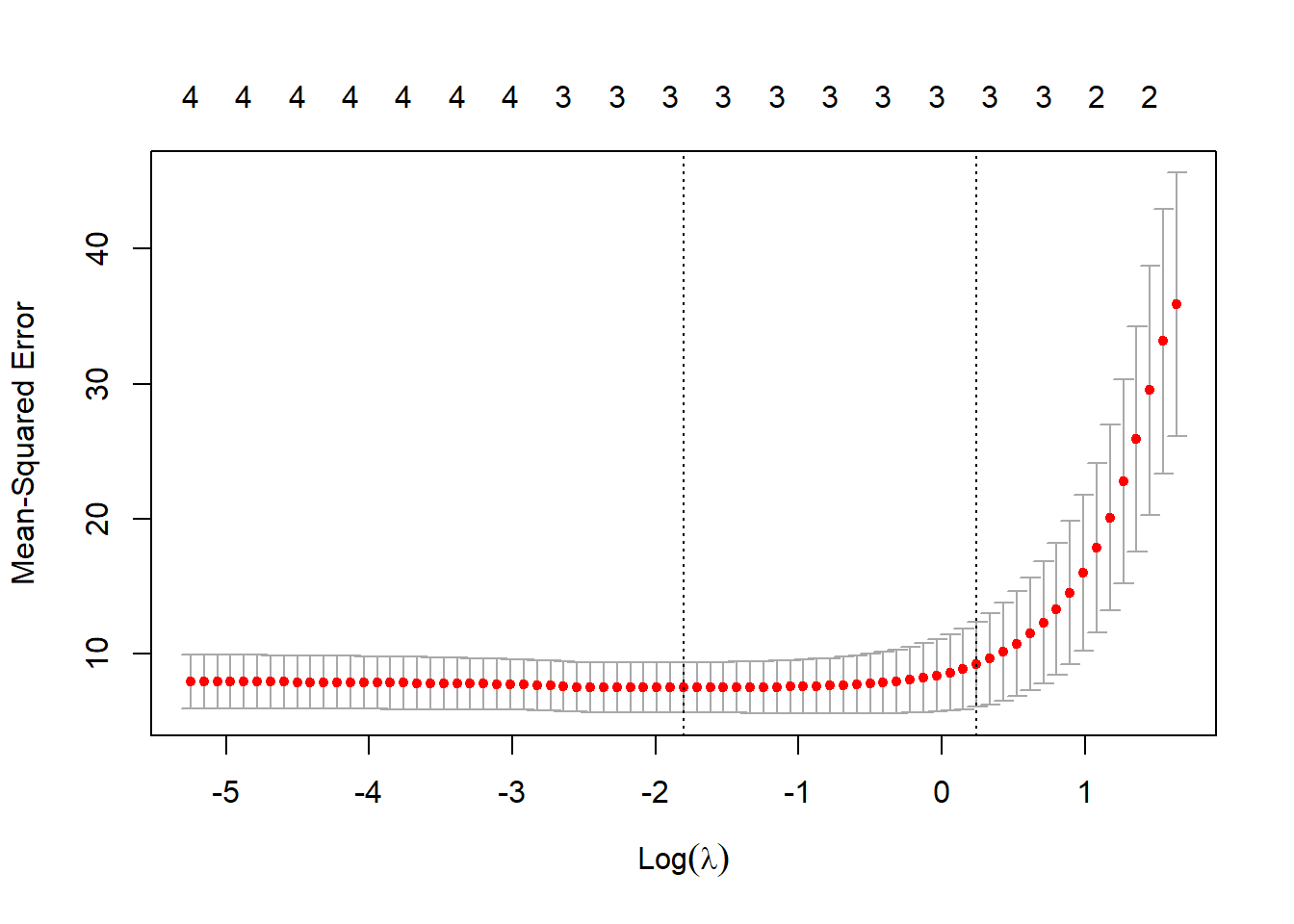# Lasso Regression

In OLS regression, the model is not penalized for its choice of weights, at all. As a result, during the training stage, if the model considers one particular feature to be particularly important, it may place a large weight on that feature i.e. derive a large value for its associated co-efficient. This can sometimes lead to overfitting, especially when it comes to small datasets with a large number of variables.

In order to reduce this potential mis-match in the size of the co-efficient associated with the different predictor variables, there is a another technique called Lasso Regression. LASSO stands for Least Absolute Shrinkage and Selection Operator. Lasso is a modification of linear regression, wherein the model is penalized by introducing the sum of absolute values of the co-efficients in the objective function. Thus, the absolute values of weight will be (in general) reduced, and many will tend to be zeros.

while Ordinary Least Squares (OLS) regression tries to find coefficient estimates that minimize the sum of squared residuals (RSS) as follows:

RSS = $$\sum (y_{i} - \hat{y})^2$$

Lasso regression tries to find coefficient estimates that minimize the following objective function:

RSS + $$\lambda\sum|\beta_{j}|$$

where: j goes from 1 to p based on the number of predictors and lambda is set greater than or equal to 0.

This second term in the equation is known as the shrinkage penalty. In lasso regression, we select a value for λ that produces the lowest possible test MSE (mean squared error).

In order to avoid penalizing the co-efficients of variables that differ widely in range of values, it is important to first scale or normalize all the variables before they can be used to fit a lasso regression model.

Now let’s look at an example of lasso regression using the in-built mtcars dataset.

# Load data
data("mtcars")
head(mtcars)
##                    mpg cyl disp  hp drat    wt  qsec vs am gear carb
## Mazda RX4         21.0   6  160 110 3.90 2.620 16.46  0  1    4    4
## Mazda RX4 Wag     21.0   6  160 110 3.90 2.875 17.02  0  1    4    4
## Datsun 710        22.8   4  108  93 3.85 2.320 18.61  1  1    4    1
## Hornet 4 Drive    21.4   6  258 110 3.08 3.215 19.44  1  0    3    1
## Hornet Sportabout 18.7   8  360 175 3.15 3.440 17.02  0  0    3    2
## Valiant           18.1   6  225 105 2.76 3.460 20.22  1  0    3    1

Here mpg is the response variable and the others are the independent variables. For our analysis, we’ll just use a subset of the predictor variables.

#define response variable
y <- mtcars$mpg #define matrix of predictor variables x <- data.matrix(mtcars[, c('hp', 'wt', 'disp', 'cyl')]) Next, we’ll use the glmnet() function to fit the lasso regression model and specify alpha = 1. To determine what value to use for lambda, we’ll perform k-fold cross-validation and identify the lambda value that produces the lowest test mean squared error (MSE). Note that the function cv.glmnet() automatically performs k-fold cross validation using k = 10 folds. library(glmnet) ## Loading required package: Matrix ## Loaded glmnet 4.1-1 #perform k-fold cross-validation to find optimal lambda value cv_model<-cv.glmnet(x, y, alpha = 1) #find optimal lambda value that minimizes test MSE best_lambda<-cv_model$lambda.min
best_lambda
##  0.1646657
#produce plot of test MSE by lambda value
plot(cv_model)The lambda value that minimizes the test MSE turns out to be 0.012.

Lastly, we can analyze the final model produced by the optimal lambda value.

We can use the following code to obtain the coefficient estimates for this model:

#find coefficients of best model
best_model<-glmnet(x, y, alpha = 1, lambda = best_lambda)
coef(best_model)
## 5 x 1 sparse Matrix of class "dgCMatrix"
##                      s0
## (Intercept) 38.18600027
## hp          -0.01675051
## wt          -3.07506079
## disp         .
## cyl         -0.92849225

No coefficient is shown for the predictor disp because the lasso regression shrunk the coefficient all the way to zero. This means it was completely dropped from the model because it wasn’t influential enough.

Note that this is a key difference between ridge regression and lasso regression. Ridge regression shrinks all coefficients towards zero, but lasso regression has the potential to remove predictors from the model by shrinking the coefficients completely to zero.

We can also use the final lasso regression model to make predictions on new observations. For example, suppose we have a new car with the following attributes:

hp: 100
wt: 2.5
disp: 125
cyl: 4

The following code shows how to use the fitted lasso regression model to predict the value for mpg for this new observation:

#define new observation
new = matrix(c(100, 2.5, 125, 4), nrow=1, ncol=4)

#use lasso regression model to predict response value
predict(best_model, s = best_lambda, newx = new)
##             1
## [1,] 25.10933

Based on the input values, the model predicts this car to have a mpg value of 25.37.

Lastly, we can calculate the R-squared of the model on the training data:

#use fitted best model to make predictions
y_predicted <- predict(best_model, s = best_lambda, newx = x)

#find SST and SSE
sst <- sum((y - mean(y))^2)
sse <- sum((y_predicted - y)^2)

#find R-Squared
rsq <- 1 - sse/sst
rsq
##  0.842224

The R-squared turns out to be 0.8484419. That is, the best model was able to explain 84.84% of the variation in the response values of the training data.